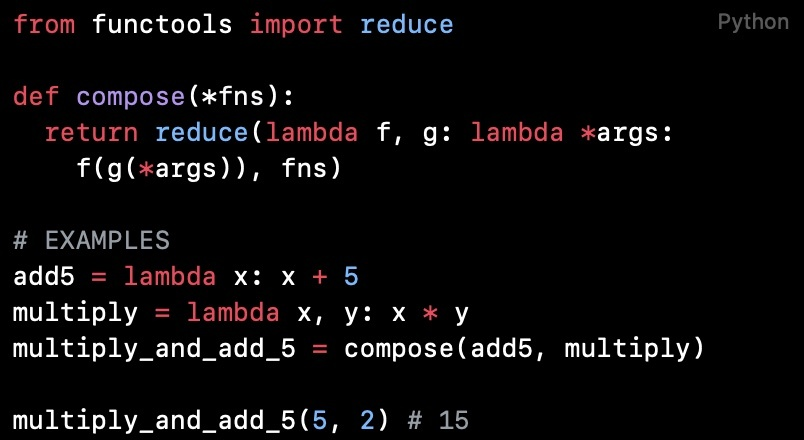# Python 代码阅读（第 43 篇）：构造组合函数FelixPython 代码阅读合集介绍：为什么不推荐Python初学者直接看项目源码

# compose

from functools import reducedef compose(*fns):  return reduce(lambda f, g: lambda *args: f(g(*args)), fns)# EXAMPLESadd5 = lambda x: x + 5multiply = lambda x, y: x * ymultiply_and_add_5 = compose(add5, multiply)multiply_and_add_5(5, 2) # 15

compose函数接收多个函数，返回将这些函数从右至左依次调用的组合函数。接收的函数中，只有最右边的函数（组合函数中第一个调用的函数）可以接收多于一个参数，其他函数只能接收一个参数。

>>> def fun(*arg):...   print(arg)...   print(type(arg))...>>> fun(1,2,3)(1, 2, 3)<class 'tuple'>

functools.reduce(function, iterable[, initializer])将两个参数的 function 从左至右积累地应用到iterable的条目，以便将该可迭代对象缩减为单一的值。 例如，reduce(lambda x, y: x+y, [1, 2, 3, 4, 5])是计算((((1+2)+3)+4)+5)的值。 左边的参数x是积累值而右边的参数y则是来自iterable的更新值。

compose函数中reduce的匿名函数lambda f, g: lambda *args: f(g(*args))可以写成如下形式：

def function1(f,g):  def function2(*args):    return f(g(*args))  return function2

>>> from functools import reduce>>> def function1(f,g):...   def function2(*args):...     return f(g(*args))...   return function2...>>> def a(arg):...   return 'a'+arg...>>> def b(arg):...   return 'b'+arg...>>> def c(arg):...   return 'c'+arg...>>> def d(arg):...   return 'd'+arg...>>> def e(arg):...   return 'e'+arg...>>> f = reduce(function1, (a,b,c,d,e))>>> print(f('f'))abcdef

# compose_right

from functools import reducedef compose_right(*fns):  return reduce(lambda f, g: lambda *args: g(f(*args)), fns)# EXAMPLESadd = lambda x, y: x + ysquare = lambda x: x * xadd_and_square = compose_right(add,square)add_and_square(1, 2) # 9## 评论